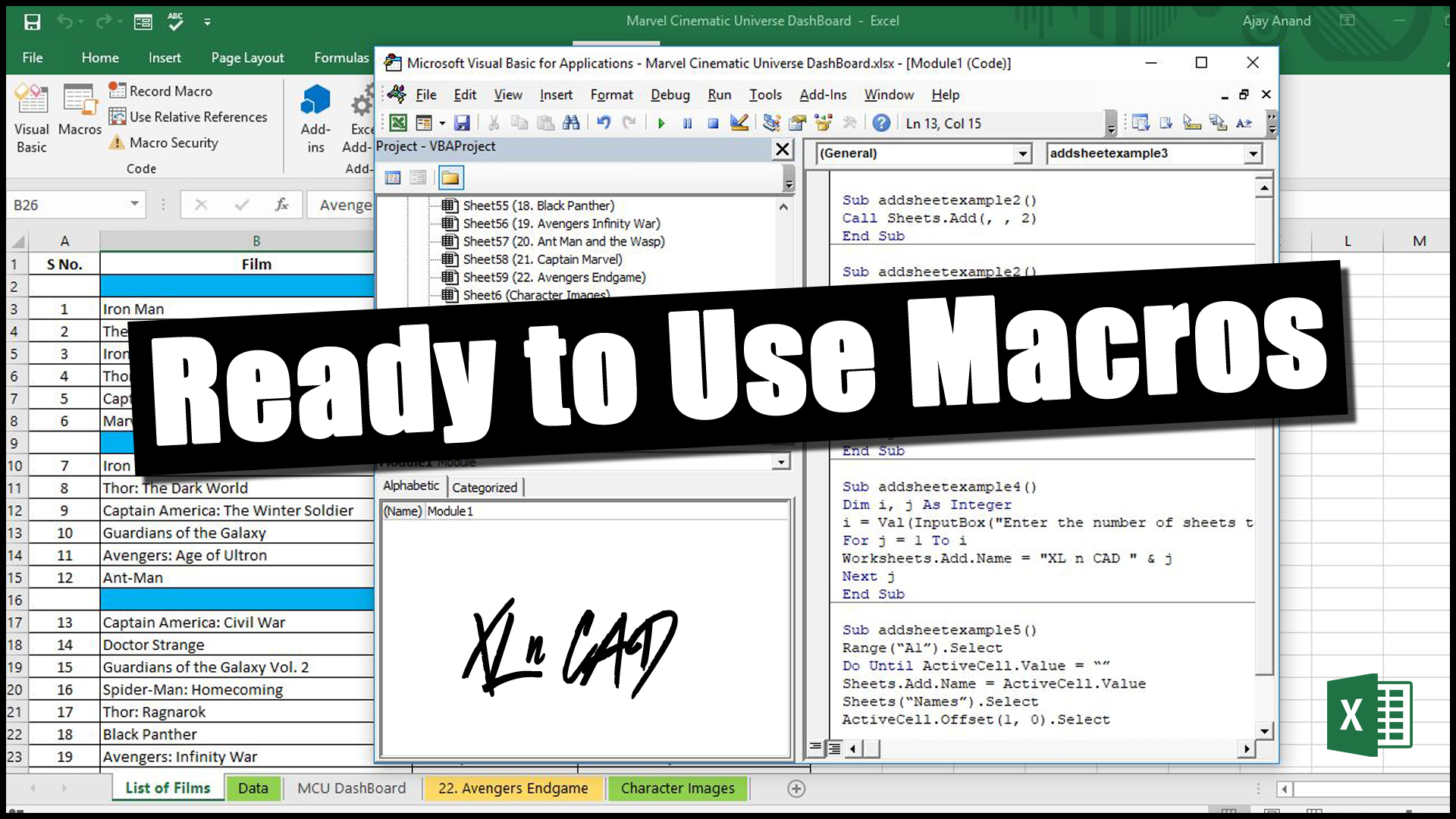# Macro to Add Multiple Sheets in Excel```Sub AddNewSheet()
End Sub```

## Add two new Worksheets into a Workbook

```Sub AddMultipleSheets()
End Sub
```

## Add Worksheets based on user input

```Sub AddSheetsUserInput()
Dim i, j As Integer
i = Val(InputBox(“Enter the number of sheets to be added”))
For j = 1 To i
Next j
End Sub```

## Add and Name the Worksheets based on User Input

`Sub AddAndNameWorksheets()Dim i, j As Integeri = Val(InputBox(“Enter the number of sheets to be added”))For j = 1 To iWorksheets.Add.Name = “XL n CAD ” & jNext jEnd Sub`

## Create worksheets from the names present in a Worksheet

```Sub CreateSheetsUsingNames()
Range(“A1”).Select
Do Until ActiveCell.Value = “”